# Top 10 Important Equations In Physics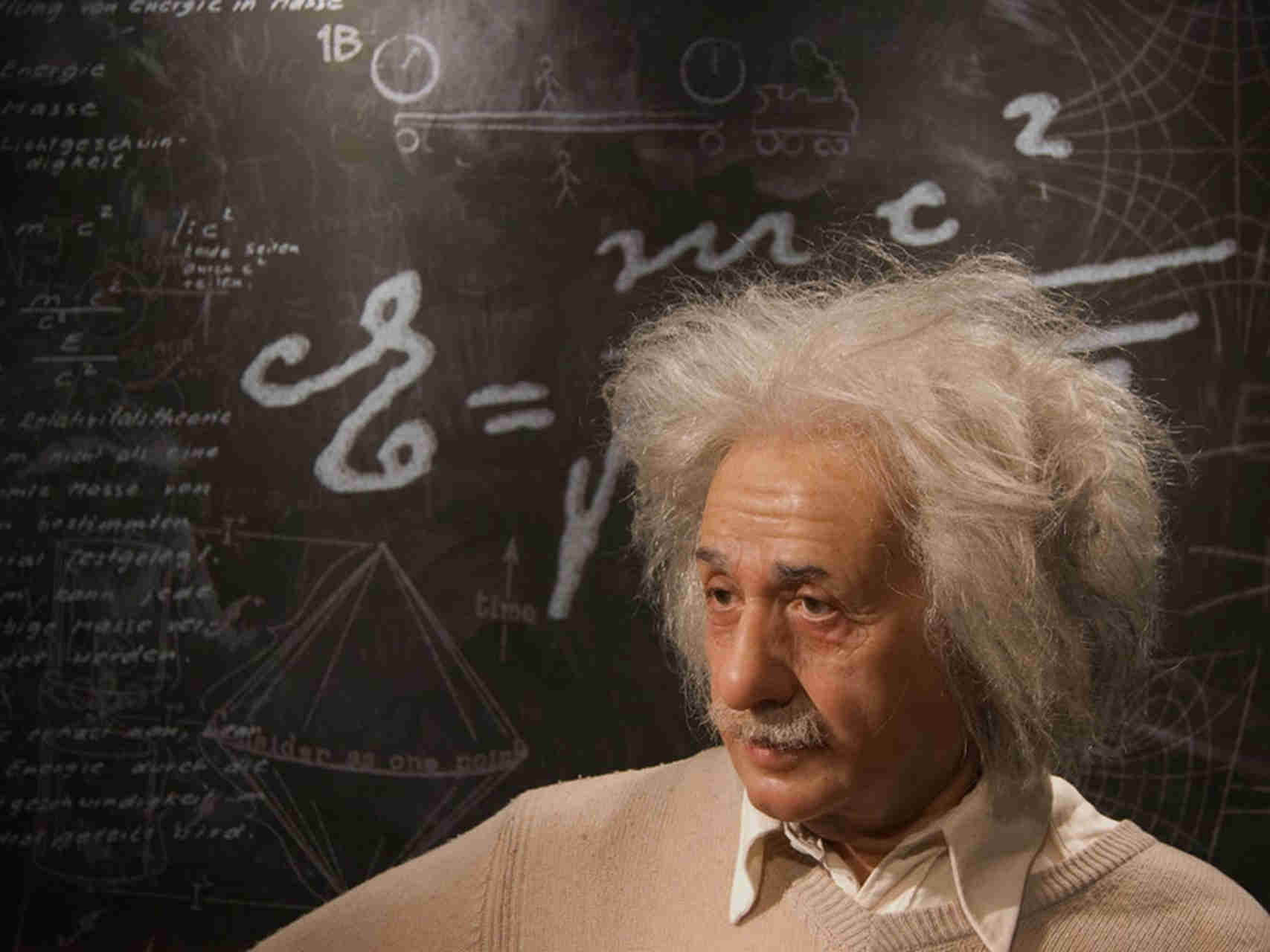These simple-looking equations went on to have great consequences and applications in science, society and technology. Let us take a look at the ten most influential formulae in physics (in no particular order) which have changed the course of history.

Second law of motion

The force, often intuitively described as push or pull, is actually the cause of acceleration in a moving particle. Without it coming from any external agency, the particle cannot undergo change in the way it goes. Newton defined the force formally in 1686 in the famous equation, F=ma.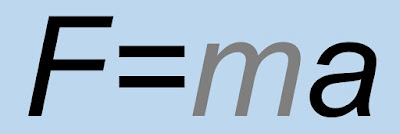It tells you how powerful an engine has to be in order to pull a car, how much thrust required to lift a rocket, how far a cannonball flies and so on. But more importantly, the equation helped debunk the Aristotelian beliefs which had remained unchallenged for thousands of years.

According to Aristotle, force is necessary to keep an object going. Why otherwise would a ball rolling on the ground eventually stop? Because, Aristotle said, it isn't pushed anymore, that's why.

Then, in the 17th century, Italian scientist Galileo Galilei explained, with experiment, "The ball stopped due to the ground being rough and had it been sufficiently smooth, the ball would roll forever. No force required!"Newton said further, that the state of rest or uniform linear motion both imply zero acceleration. Thus, the particle will remain in place or keep going at the same rate and it will maintain itself in the state in which it's been until of course when acted upon by external force.

Energy-mass relation

It followed from the special theory of relativity that mass and energy are both but different manifestations of the same thing. The mass of a body is a measure of its energy content. If 1 gram of mass is converted into energy, it'd be 90 trillion Joules. This is equal to the energy emitted by a 100 watt light bulb for 30,000 years!

It is important to understand that Einstein's most famous equation is not his major work. The formula is just so well-known because of its association with the atomic bomb. Einstein himself had said, "If I had foreseen Hiroshima and Nagasaki, I would have torn up my formula in 1905," despite him having played a minor role in the Manhattan Project.

Uncertainty principle

Formulated by Werner Heisenberg in 1927, uncertainty principle is one of the cornerstones of quantum mechanics. The equation single-handedly ended the classical determinism, meaning, that in the realm of infinitesimal atoms, chance has its play and the drama of existence is not absolutely predestined in character.

In its most familiar form, it says that the more precise the measurement of position, the more imprecise the measurement of momentum, and vice-versa. Thus, one can never know with perfect accuracy both of those two important factors which determine the movement of one of the smallest particles, its position and its velocity, at the same instant.The uncertainty principle was immediately rejected by leading physicists of the time, including Albert Einstein. There, Niels Bohr did try his best to convince Einstein that the uncertainty relation is fundamental law in physics. Einstein still refused, and they agreed to disagree. By 1933, the political situation became much worse in Germany, and Einstein moved to the United States.

In 1954, Heisenberg visited Einstein's house in Princeton. They talked only about physics, but Einstein's position on the principle hadn't changed. In 1955, Einstein passed away leaving Werner Heisenberg disheartened that he had failed to get Einstein's endorsement of his uncertainty relation.

Although Einstein and others objected to Heisenberg's and Bohr's views, even Einstein had to admit that they were indeed a logical consequence of quantum mechanics. But for Einstein, something still was missing and the quantum mechanics was incomplete, "I am convinced that god does not throw dice," he claimed metaphorically.

Heisenberg, supported by Bohr, Pauli, Schrödinger and others, maintained until his death that quantum uncertainty is not inaccuracy of the measurement, it is inherent in quantum phenomena. It leads to probabilistic and not deterministic outcomes.

In 1831, as the story is usually told, the prime minister or some other senior politician was given a demonstration of electromagnetic induction by Faraday. When asked, “What good is it?” Faraday replied: “What good is a newborn baby?” Fifty years passed before electric power really took off as envisioned by Faraday.Generators and motors both make use of Faraday's Law. The equation by Maxwell became the foundation of power generation hence making Faraday the father of electricity. Maxwell said of Faraday, "He is, and must always remain, the father of that enlarged science of electromagnetism."

Dirac equation

Symmetry is the keyword of physics and Dirac used it perfectly in 1928. He developed an equation that explained spin number as a consequence of the union of quantum mechanics and special relativity. The equation also predicted the existence of anti-matter, previously unsuspected and unobserved, and which was experimentally discovered in 1932.This accomplishment has been described on par with the works of Newton, Maxwell, and Einstein before him. Dirac even speculated that there may also be mirror universe of anti-particles, thus becoming a source of inspiration for science-fiction writers. Dirac was also equally famous for his contribution to quantum electrodynamics, which described how electric and magnetic forces would work on the scale of things smaller than atoms.

Law of entropy

The famous inequality which says that when energy changes from one form to another form, or when matter moves freely, the disorder in a closed system increases. According to renowned astronomer Arthur Eddington, "The law that entropy always increases, holds, I think, the supreme position among the laws of nature."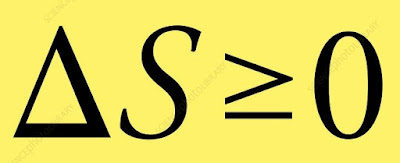The concept of the second law of thermodynamics applies not only to internal combustion engines used in our cars, motorcycles, ships and airplanes but also to explain the processes of life, when considered in terms of cyclic processes.

The second law also has profound consequences for the universe in large scale. Imagine being shown a video clip of a cup being dropped and breaking. You'd clearly be able to tell whether the video was being played backward or forward, from the flow of entropy.

Similarly, if the movie of our universe is played backwards, the universe would be getting more and more ordered, like the cup, and when played forward, we'd expect it getting disordered, like the pieces of broken cup.

Einstein field equationsEinstein's equations led to the fusion of the three dimensions of space and the one dimension of time into a single four-dimensional spacetime. The expression on the left hand side of the equation represents the curvature of spacetime. The expression on the right is the energy density of spacetime. The equation dictates how energy determines the curvature of space and time.

The cosmological constant term (Λ) was introduced by Einstein to allow for a universe that is not expanding or contracting. This effort was unsuccessful because in 1929, astronomer Edwin Hubble discovered evidences for an expanding universe. Einstein was invited by Hubble to see for himself that the universe indeed was changing.As a result, Einstein abandoned the cosmological constant in the equation, calling it the biggest blunder he ever made. So from the 1930s until the late 1990s, most physicists assumed the cosmological constant to be equal to zero. But, recently improved astronomy techniques have found that the expansion of the universe is accelerating implying the non-zero value of the constant.

Why are the Einstein field equations important in physics? Firstly, because they unify the two concepts of space and time, previously considered separate by the limitations of our intuition, into one spacetime. Just like Maxwell had unified electricity and magnetism into electromagnetism in the 19th century.

Secondly, they describe – not the force – but the fundamental "interaction" of gravitation as a result of spacetime being curved by energy (mass too is energy from Einstein's energy-mass equivalence).

Although Newton did give the formula to calculate the magnitude of gravitational force between any two bodies of mass separated by a distance, he didn't quite explain the cause of gravitation in the first place.

Wave equationThe single-dimensional wave equation has a scalar function (u) of one space variable and one time variable since waves propagate in space, and in time also. This equation was first written by French mathematician Jean le Rond d'Alembert, hence it's sometimes also called the d'Alembert's equation. Swiss mathematician and physicist Leonhard Euler wrote it in three dimensions in 1707.

We are constantly surrounded by waves, whether perceptible to us or not, they are always there. Like when you play a guitar or drop a stone into a pond. The wave equation isn't as elegant as others on this list but it is groundbreaking as it's been applied to sound waves (and instruments), waves in fluids, waves in earthquakes, light waves, quantum mechanics and general relativity.

Planck's equation

This formula is responsible for the birth of quantum mechanics, also television and solar cells. Leading German physicist of the time Max Planck postulated in 1900, that energy was quantised and could be emitted or absorbed only in integral multiples of a small unit, which he called "energy quantum".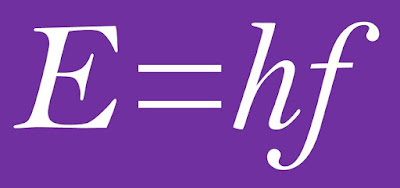Einstein extended Planck's idea in 1905 when he introduced the concept of "light quantum", the particle of light, or photon. Thus, the electromagnetic radiation wasn't continuous like a wave but isolated in the packets of light, Einstein proposed.

Planck had simply introduced the equation as a trick to solve a problem with black body radiation, but Einstein envisioned it to be more. In 1887, experimenter Heinrich Hertz stumbled upon the photoelectric effect for the first time; the emission of electrons when light of specific frequency hit a material.

The phenomenon of photoelectric effect remained largely unexplained, even with the wave theory of light, until the arrival of Planck-Einstein relation in 1905. Einstein described it in terms of particle-particle interaction between the photon and electron. He said, "...below some critical frequency, no photon has enough energy to knock an electron free."

This means that if a photosensitive material requires photons of blue light to emit the electrons, which is the characteristic of the material, then the photons of green or yellow light won't be able to knock the electrons out of the material.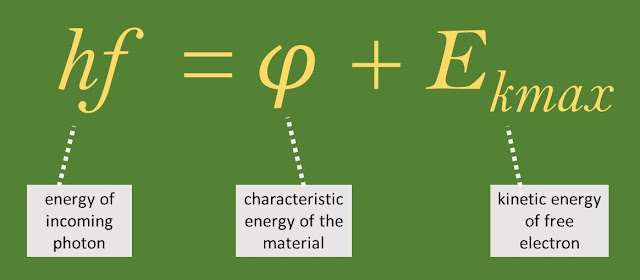The characteristic energy or work-function of the material is absorbed, to loosen the bonds, and then the remainder of the energy is observed as kinetic energy of the free electron. Einstein's clarification was consistent with the law of conservation of energy. He was recognized with Nobel Prize in physics for his explanation of the photoelectric effect (and not for energy-mass relation or relativity).

Planck said his introduction of "quantum" in 1900 was an act of desperation but when Einstein adopted it and gave it meaning, a whole new debate had started and the old laws were swept away within a decade or so. Einstein who himself was accountable for it refused to endorse the new quantum revolution.

The discovery by Planck and Einstein became the basis of all twentieth-century physics, without which, it would not have been possible to establish a workable theory of molecules and atoms and the energy processes that govern their transformations.

Schrödinger's equation

In his 1924 doctoral thesis, French physicist Louis de Broglie proposed, that just like light has both wave and particle properties, electrons must also possess wave-like properties, in order to support the energy-matter symmetry. Two years later, in 1926, Austrian scientist Erwin Schrödinger published an equation, describing how the matter wave should evolve in space and in time.Just like Newton's equations are used to calculate how a football behaves when kicked, you use the Schrödinger's equation to calculate the behaviour of electron in the orbit of an atom. More generally, it is used for many calculations in quantum mechanics and is also fundamental to much of the modern technology, from lasers to transistors, and the future development of quantum computers.